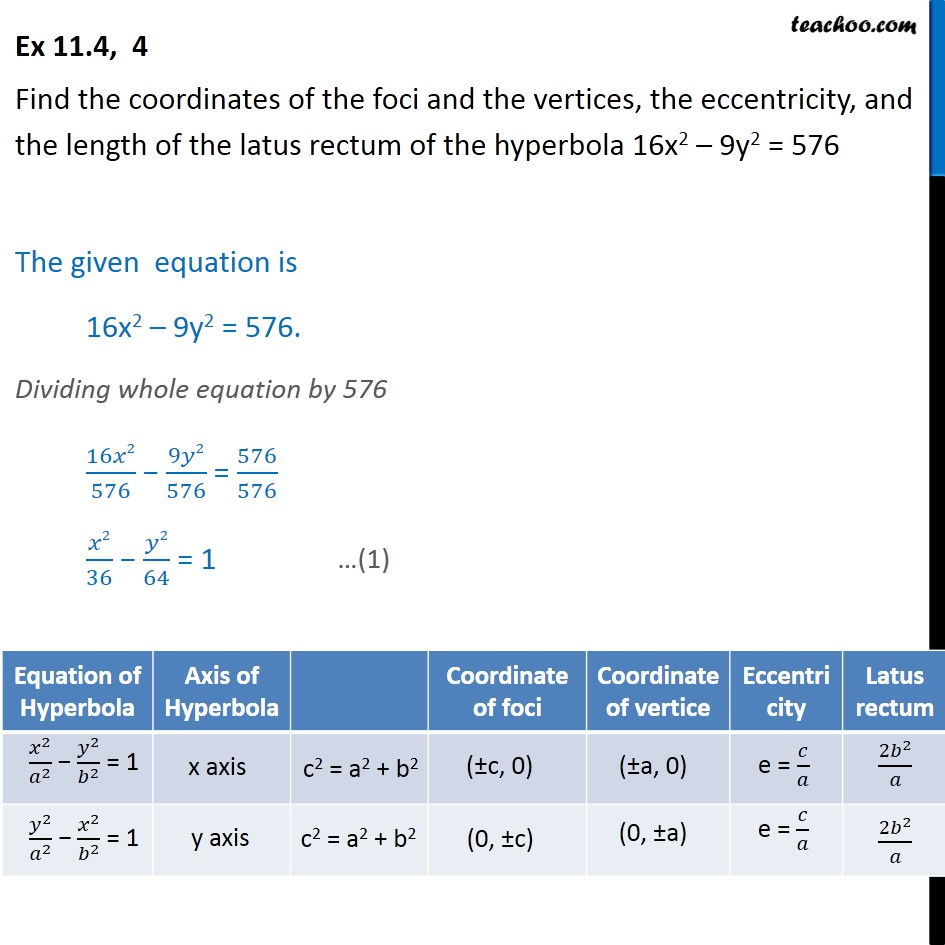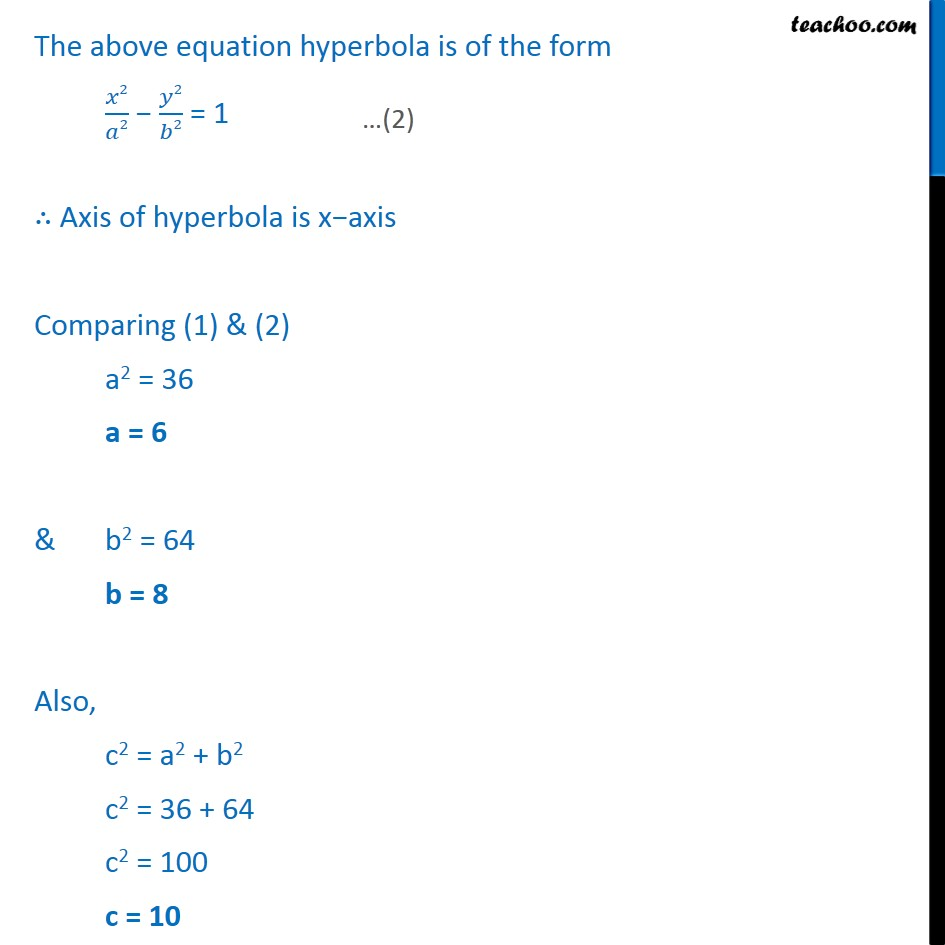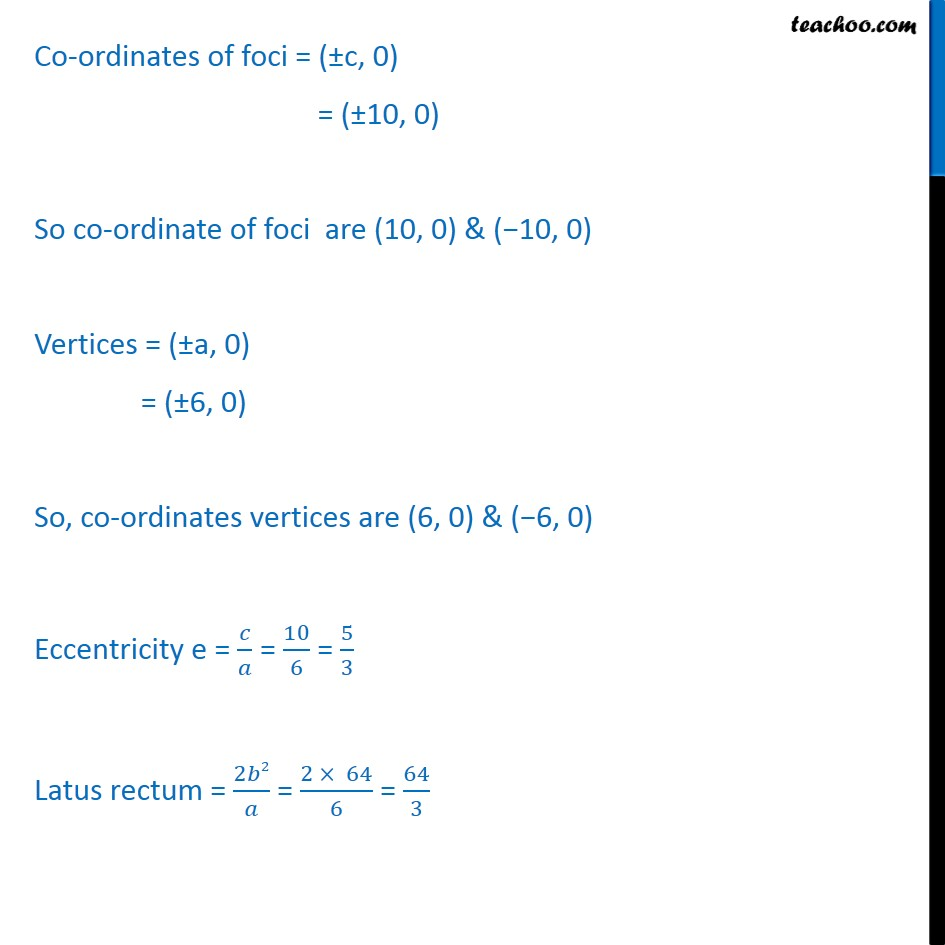Ex 10.4

Chapter 10 Class 11 Conic Sections
Serial order wiseLearn in your speed, with individual attention - Teachoo Maths 1-on-1 Class

### Transcript

Ex 10.4, 4 Find the coordinates of the foci and the vertices, the eccentricity, and the length of the latus rectum of the hyperbola 16x2 9y2 = 576 The given equation is 16x2 9y2 = 576. Dividing whole equation by 576 16 2 576 9 2 576 = 576 576 2 36 2 64 = 1 The above equation hyperbola is of the form 2 2 2 2 = 1 Axis of hyperbola is x axis Comparing (1) & (2) a2 = 36 a = 6 & b2 = 64 b = 8 Also, c2 = a2 + b2 c2 = 36 + 64 c2 = 100 c = 10 Co-ordinates of foci = ( c, 0) = ( 10, 0) So co-ordinate of foci are (10, 0) & ( 10, 0) Vertices = ( a, 0) = ( 6, 0) So, co-ordinates vertices are (6, 0) & ( 6, 0) Eccentricity e = = 10 6 = 5 3 Latus rectum = 2 2 = 2 64 6 = 64 3